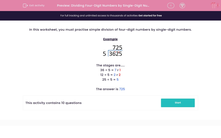# Dividing Four-Digit Numbers by Single-Digit Numbers (2)

In this worksheet, students improve their fluency at dividing four-digit numbers by single-digit numbers.This content is premium and exclusive to EdPlace subscribers.Key stage:  KS 2

Curriculum topic:   Maths and Numerical Reasoning

Curriculum subtopic:   Mixed Problems

Difficulty level:#### Worksheet Overview

In this worksheet, you must practise simple division of four-digit numbers by single-digit numbers.

ExampleThe stages are.....

36 ÷ 5 = 7 r 1

12 ÷ 5 = 2 r 2

25 ÷ 5 = 5